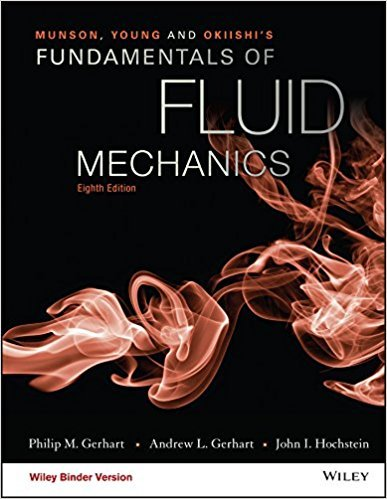×
Get Full Access to Fundamentals Of Fluid Mechanics - 8 Edition - Chapter 5.2.4 - Problem 5.85
Get Full Access to Fundamentals Of Fluid Mechanics - 8 Edition - Chapter 5.2.4 - Problem 5.85

×

# The hydraulic turbine shown in Fig. P5.85 has a 10 C waterflow rate of 36.4 m3/s, anISBN: 9781119080701 456

## Solution for problem 5.85 Chapter 5.2.4

Fundamentals of Fluid Mechanics | 8th Edition

• Textbook Solutions
• 2901 Step-by-step solutions solved by professors and subject experts
• Get 24/7 help from StudySoup virtual teaching assistantsFundamentals of Fluid Mechanics | 8th Edition

4 5 1 253 Reviews
25
2
Problem 5.85

The hydraulic turbine shown in Fig. P5.85 has a 10 C waterflow rate of 36.4 m3/s, an inlet radius R1 = 1.0 m, an outlet radiusR2 = 0.50 m, a blade depth (perpendicular to paper) h = 0.50 m,and a rotational speed N = 360 rpm; V1 = 50 m/s, V2 = 30 m/s,1 = 13.4, and 2 = 40. Calculate the power transferred by thefluid to the rotor, the inlet relative velocity W1, and the directionW1 makes with the radius at the inlet.

Step-by-Step Solution:
Step 1 of 3

2/23/16: The Nervous System Control Systems in Animals Physiology of animals is controlled by two main systems: 1. Endocrine 2. Nervous Nervous System ● Rapid responses via electrical signals → works quicker than you even realize. ● Interprets information about your body and the environment, decides whether and how to respond. ● The control center. ● Made of neuron/nerve and support/glial cells. ● Two subdivisions: ○ CNS: Brain and spinal cord. ○ PNS: Neurons outside of the CNS. The Central Nervous System Has two parts: 1. Brain 2. Spinal cord 3. Interneurons: Link b/w sensory and motor neurons. Receives and integrates information from the body and directs an appropriate output response. **The spinal column refers to the spine and the nerve cord is the “whole package.” Brain: the control center for the entire body ● Physical movement, emotions, thoughts, endocrine signals, individuality, learning, memories, language, sensations. Brain Anatomy Cerebrum: The largest part; anterior in skull; two hemispheres; several regions. ● Frontal, parietal, occipital, temporal lobes. Cerebellum: Posterior in skull; beneath the cerebrum; muscle coordination. ● Only 10% of the brain mass, but contains ½ of the brain’s neurons. The brain is gray, due to the things wrapped around the neurons. ● Two hemispheres joined by a nerve bundle in the center which is called the corpus callosum.

Step 2 of 3

Step 3 of 3

##### ISBN: 9781119080701

The answer to “The hydraulic turbine shown in Fig. P5.85 has a 10 C waterflow rate of 36.4 m3/s, an inlet radius R1 = 1.0 m, an outlet radiusR2 = 0.50 m, a blade depth (perpendicular to paper) h = 0.50 m,and a rotational speed N = 360 rpm; V1 = 50 m/s, V2 = 30 m/s,1 = 13.4, and 2 = 40. Calculate the power transferred by thefluid to the rotor, the inlet relative velocity W1, and the directionW1 makes with the radius at the inlet.” is broken down into a number of easy to follow steps, and 84 words. The full step-by-step solution to problem: 5.85 from chapter: 5.2.4 was answered by , our top Science solution expert on 03/16/18, 03:21PM. This textbook survival guide was created for the textbook: Fundamentals of Fluid Mechanics, edition: 8. Since the solution to 5.85 from 5.2.4 chapter was answered, more than 255 students have viewed the full step-by-step answer. This full solution covers the following key subjects: . This expansive textbook survival guide covers 112 chapters, and 1357 solutions. Fundamentals of Fluid Mechanics was written by and is associated to the ISBN: 9781119080701.

Unlock Textbook Solution